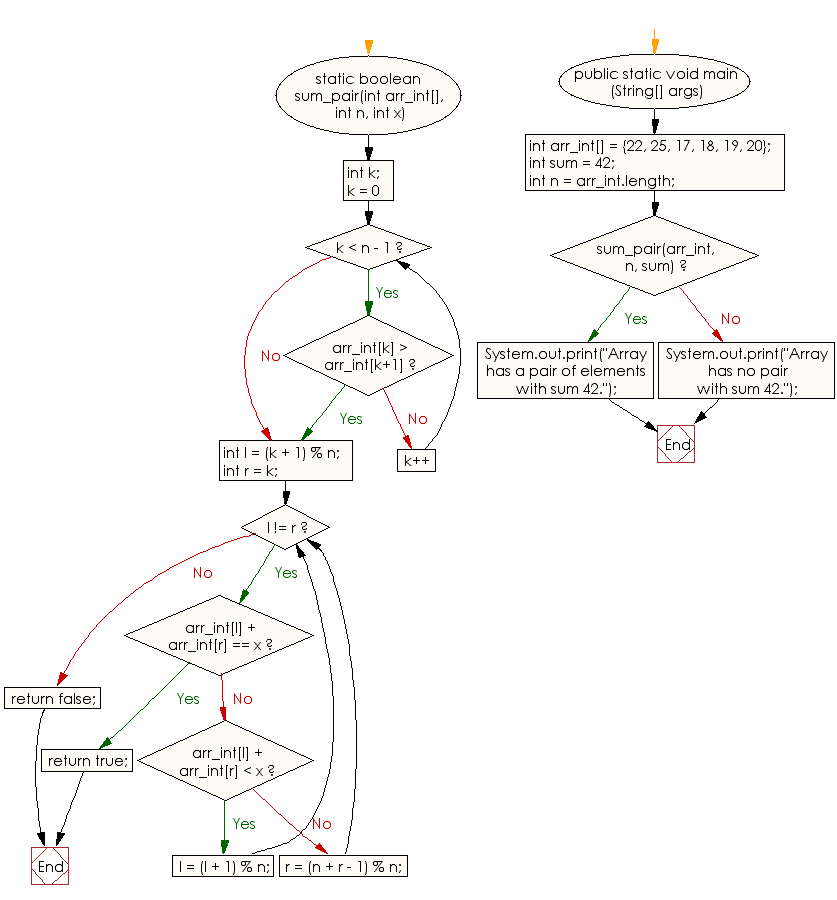﻿ Java exercises: Check whether there is a pair with a specified sum of a given sorted and rotated array - w3resource# Java Array Exercises: Check whether there is a pair with a specified sum of a given sorted and rotated array

## Java Array: Exercise-46 with Solution

Write a Java program to check whether there is a pair with a specified sum of a given sorted and rotated array.

Sample Solution:

Java Code:

``````public class Main
{
static boolean sum_pair(int arr_int[],
int n, int x)
{
int k;
for (k = 0; k < n - 1; k++)
if (arr_int[k] > arr_int[k+1])
break;

int l = (k + 1) % n;

int r = k;

while (l != r)
{
if (arr_int[l] + arr_int[r] == x)
return true;
if (arr_int[l] + arr_int[r] < x)
l = (l + 1) % n;

else
r = (n + r - 1) % n;
}
return false;
}

public static void main (String[] args)
{
int arr_int[] = {22, 25, 17, 18, 19, 20};
int sum = 42;
int n = arr_int.length;

if (sum_pair(arr_int, n, sum))
System.out.print("Array has a pair of elements with sum 42.");
else
System.out.print("Array has no pair with sum 42.");
}
}
```
```

Sample Output:

```
Array has a pair of elements with sum 42.
```

Flowchart:Visualize Java code execution (Python Tutor):

Java Code Editor:

Improve this sample solution and post your code through Disqus

What is the difficulty level of this exercise?

﻿

## Java: Tips of the Day

Array vs ArrayLists:

The main difference between these two is that an Array is of fixed size so once you have created an Array you cannot change it but the ArrayList is not of fixed size. You can create instances of ArrayLists without specifying its size. So if you create such instances of an ArrayList without specifying its size Java will create an instance of an ArrayList of default size.

Once an ArrayList is full it re-sizes itself. In fact, an ArrayList is internally supported by an array. So when an ArrayList is resized it will slow down its performance a bit as the contents of the old Array must be copied to a new Array.

At the same time, it's compulsory to specify the size of an Array directly or indirectly while creating it. And also Arrays can store both primitives and objects while ArrayLists only can store objects.

Ref: https://bit.ly/3o8L2KH